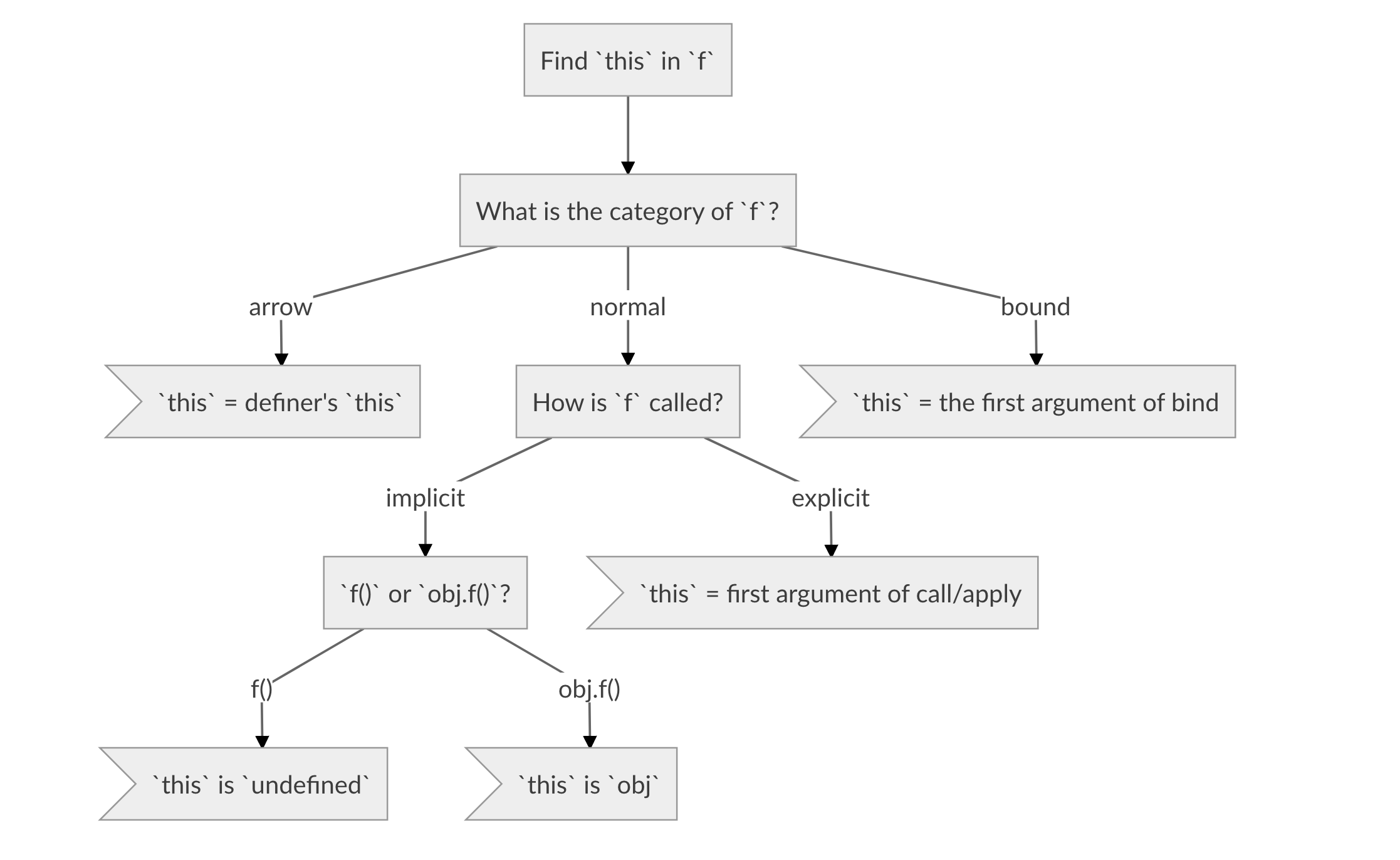YCM Jason

# Let me explain to you what is `this`. (Javascript)

Original post: https://www.ycmjason.com/blog/2018/06/15.html

`this` in Javascript is the probably most magical keyword in the programming world. It's unpredictable nature has reached to an unprecedented level.However, it is essential to understand it fully if you wish to become a master of Javascript. So let me try to explain you what is `this`. (if it doesn't work, well, at least I tried.)

## # Functions

Starting with functions. In this article, I would like to put functions into 3 different categories.

1. Normal functions
2. Arrow functions
3. Bound functions

### # Normal functions

I define normal functions as any function created with...

``````// function declaration
function magic() {
...
}

// function expression
const magic = function() {
...
};

// (or if you hate your life)
// function constructor
const magic = new Function('...');
``````

### # Arrow functions

Arrow functions are basically the ES6 arrow functions:

``````const magic = () => {
...
};
``````

### # Bound functions

Bound functions can be created by calling `Function.prototype.bind` on a normal functions.

``````// magic is created with function declaration/expression/constructor
const bound = magic.bind(...);
``````## # Ways to call a function

Now let's say we have a function `f` (any category). There are 2 ways to call it.

1. Implicit (direct) calls
2. Explicit calls

### # Implicit (direct) calls

Implicit (direct) calls are boring:

``````/* f is defined */

// direct call
f();

// or attach it to an object and call it
const obj = {};
obj.fn = f;
obj.fn();
``````

### # Explicit call

Explicit calls are more interesting. You can call your function with `Function.prototype.call` or `Function.prototype.apply`.

``````/* f is defined */

// Function.prototype.call
f.call(...);

// Function.prototype.apply
f.apply(...);
``````## # Quick recap

Let's do a quick recap, we have 3 categories of functions:

1. Normal functions - created with function declaration/expression/constructor
2. Arrow functions - `() => {...}`
3. Bound functions - created with `f.bind(...)`

And 2 ways to call a function:

1. Implicit (direct) calls - `f()` or `obj.f()`
2. Explicit calls - `f.call(...)` or `f.apply(...)`

This means we have 6 different scenarios.

1. Normal functions + Implicit (direct) calls
2. Normal functions + Explicit calls
3. Arrow functions + Implicit (direct) calls
4. Arrow functions + Explicit calls
5. Bound functions + Implicit (direct) calls
6. Bound functions + Explicit callsDon't panic, it is not that scary.

In fact, arrow functions and bound functions do not care about implicit/explicit calls. So this reduces down to only 4 scenarios:

1. Normal functions + Implicit (direct) calls
2. Normal functions + Explicit calls
3. Arrow functions
4. Bound functions

## # Procedure to find `this`Below is the procedure to find the binding of `this` in function `f`:## # Exercises!

Given `magic` defined as follows:

``````'use strict';

const magic = function() {
// a. what is `this`?
console.log(this);

const cool = () => {
// b. what is `this`?
console.log(this);
};
cool();
};

// QUESTION 1
magic();

// QUESTION 2
const apple = { name: 'apple' };
apple.magic = magic;
apple.magic();

// QUESTION 3
const orange = { name: 'orange' };
magic.call(orange);
``````

### # QUESTION 1.a

Following flow chart, we want to find `this` in `magic`.

1. Category of `magic` is normal function
2. `magic` is called implicitly (directly)
3. `magic` is called with `magic()`
4. So `this` = `undefined`!!!

### # QUESTION 1.b

Following flow chart, we want to find `this` in `cool`.

1. Category of `cool` is arrow function
2. From QUESTION 1.b, we know `magic`'s `this` is `undefined`
3. `cool`'s definer is `magic`
4. So `this` = `magic`'s `this` = `undefined`!

## # Lazy lecturer

The remaining questions, QUESTION 2.a, 2.b, 3.a and 3.b, are trivial with my flow chart. So I will leave them as an exercise for you all.

https://repl.it/@ycmjason/What-is-this

Click run and you will see the answer in order (1.a, 1.b, 2.a, 2.b, 3.a, 3.b).

## # Note

• There is no "bound arrow function". `(() => {...}).bind(...)` is still the original arrow function.
• For implicit calling, only the shape (`f()` or `obj.f()`) matter. It doesn't matter where `f` comes from. Consider the following code:
``````const f = obj.f; // obj.f is a normal function
f(); // `this` in the body of `f` is `undefined`!!! not `obj`!!!
``````

• 16 July 2018: Thanks to @joshcheek for reminding me the correct binding of `this` of arrow functions!